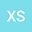•In this paper, we study the following Cauchy problem \begin{equation*} \left\{ \begin{array}{lll} iu_t=\Delta u+2uh’(|u|^2)\Delta h(|u|^2)+F(|u|^2)u\mp A[h(|u|^2]^{2^*-1}h’(|u|^2)u,\ x\in \mathbb{R}^N, \ t>0,\\ u(x,0)=u_0(x),\quad x\in \mathbb{R}^N. \end{array}\right. \end{equation*} Here $h(s)$ and $F(s)$ are some real-valued functions, $h(s)\geq 0$ and $h’(s)\geq 0$ for $s\geq 0$, $N\geq 3$, $A>0$. Besides obtaining sufficient conditions on the blowup in finite time and global existence of the solution, we get Morawetz type estimates and weighted spacetime bounds for the global solution based on pseudoconformal conservation law, we also establish a sharp threshold which gives the watershed for initial data $u_0$ making the solution blow up.